## Histogram: Study the shape

A histogram can be created using software such as SQCpack. How would you describe the shape of the histogram?

Bell-shaped: A bell-shaped picture, shown below, usuallypresents a normal distribution.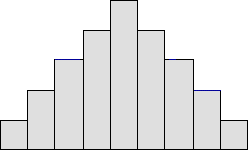Bimodal: A bimodal shape, shown below, has two peaks. This shape may show that the data has come from two different systems. If this shape occurs, the two sources should be separated and analyzed separately.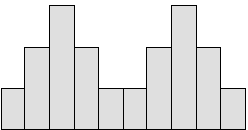Skewed right: Some histograms will show a skewed distribution to the right, as shown below. A distribution skewed to the right is said to be positively skewed. This kind of distribution has a large number of occurrences in the lower value cells (left side) and few in the upper value cells (right side). A skewed distribution can result when data is gathered from a system with has a boundary such as zero. In other words, all the collected data has values greater than zero.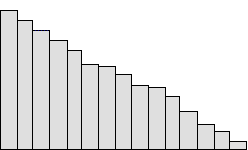Skewed left: Some histograms will show a skewed distribution to the left, as shown below. A distribution skewed to the left is said to be negatively skewed. This kind of distribution has a large number of occurrences in the upper value cells (right side) and few in the lower value cells (left side). A skewed distribution can result when data is gathered from a system with a boundary such as 100. In other words, all the collected data has values less than 100.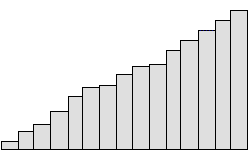Uniform: A uniform distribution, as shown below, provides little information about the system. An example would be a state lottery, in which each class has about the same number of elements. It may describe a distribution which has several modes (peaks). If your histogram has this shape, check to see if several sources of variation have been combined. If so, analyze them separately. If multiple sources of variation do not seem to be the cause of this pattern, different groupings can be tried to see if a more useful pattern results. This could be as simple as changing the starting and ending points of the cells, or changing the number of cells. A uniform distribution often means that the number of classes is too small.Random: A random distribution, as shown below, has no apparent pattern. Like the uniform distribution, it may describe a distribution that has several modes (peaks). If your histogram has this shape, check to see if several sources of variation have been combined. If so, analyze them separately. If multiple sources of variation do not seem to be the cause of this pattern, different groupings can be tried to see if a more useful pattern results. This could be as simple as changing the starting and ending points of the cells, or changing the number of cells. A random distribution often means there are too many classes.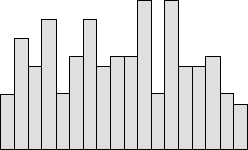Follow these steps to interpret histograms.